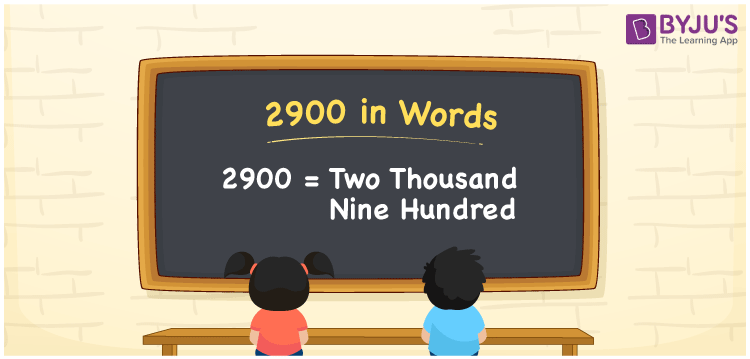# 2900 in Words

2900 in words is written as Two thousand nine hundred. In both the International System of Numerals and the Indian System of Numerals, 2900 is written as Two thousand nine hundred. The number 2900 is a Cardinal Number as it represents some quantity. For example, “the dress costs 2900 rupees”.

 2900 in words Two thousand nine hundred Two thousand nine hundred in Number 2900

## 2900 in English Words

2900 in English words is read as “Two thousand nine hundred.”## How to Write 2900 in Words?

To write 2900 in words, we shall use the place value chart. In the place value chart, put 2 in the thousands, 9 in the hundreds, and 0 in the tens and the ones place respectively. Let us make a place value chart to write the number 2900 in words.

 Thousands Hundreds Tens Ones 2 9 0 0

Thus, we can write the expanded form as

2 × Thousand + 9 × Hundred + 0 × Ten + 0 × One

= 2 × 1000 + 9 × 100 + 0 × 10 + 0 × 1

= 2000 + 900 + 0 + 0

= 2900

= Two thousand nine hundred.

2900 is the natural number that is succeeded by 2899 and preceded by 2901.

2900 in words – Two thousand nine hundred

• Is 2900 an odd number? – No
• Is 2900 an even number? – Yes
• Is 2900 a perfect square number? – No
• Is 2900 a perfect cube number? – No
• Is 2900 a prime number? – No
• Is 2900 a composite number? – Yes

## Frequently Asked Questions on 2900 in Words

### How to write 2900 in words?

2900 in words is written as Two thousand nine hundred.

### How to write 2900 in words in the International and Indian System of Numerals?

In both, the system of numerals, 2900 in words, is written as Two thousand nine hundred.

### How to write 2900 in a place value chart?

In the place value chart, write 2 in the thousands, 9 in the hundreds, and 0 in the tens and the ones, respectively.